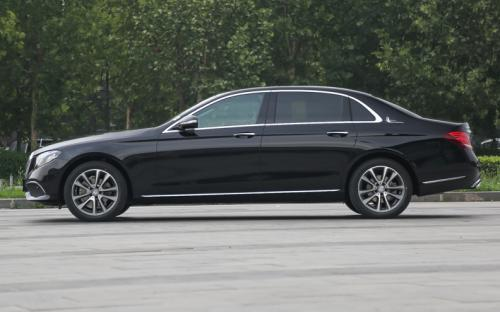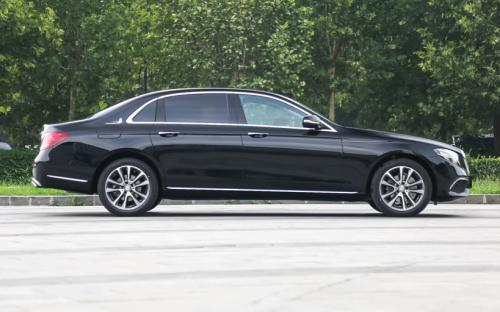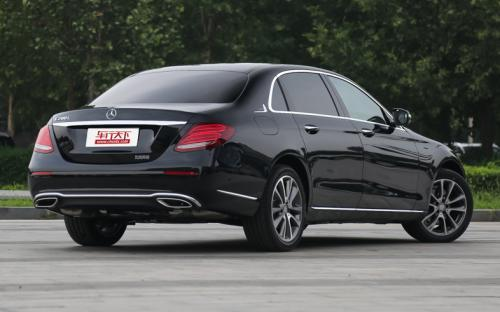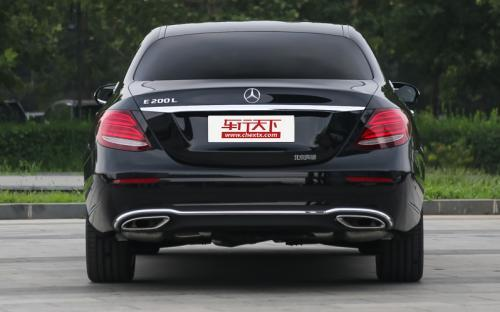### 北京奔驰 奔驰E级0 种颜色可选2016款最低售价：43.68 万元起

5065(mm)1860(mm)1467(mm)##### 配置亮点：
• 胎压监测装置

• ISOFIX儿童座椅接口

• 车身稳定控制(ESC/ESP/DSC等)

• 电动天窗

• 定速巡航

• 后倒车雷达

• 真皮座椅

• GPS导航系统

• 氙气大灯

• 后视镜加热

• 提交
2016款 E 300 L 运动时尚型 (109张)
• 2016款 E 300 L 运动时尚型 (109张)
• 2016款 E 300 L 豪华型 (325张)
• 北京奔驰 奔驰E级 绕车实拍• 北京奔驰 奔驰E级 在售车型

排量 车型 厂商指导价 本地最低报价 购车工具
2.0L
E 200 L 停产在售 9挡手自一体
43.68万
E 200 L 运动型 停产在售 9挡手自一体
43.68万
E 300 L 时尚型 停产在售 9挡手自一体
47.48万
E 300 L 运动时尚型 停产在售 9挡手自一体
47.48万
E 300 L 豪华型 停产在售 9挡手自一体
49.98万
E 300 L 运动豪华型 停产在售 9挡手自一体
49.98万

北京奔驰 奔驰E级 经销商

查看更多 >>

### 北京奔驰 奔驰E级 动力加速

奔驰E级 0-100公里加速时间分布在 6.9-8.6秒 属于 高性能级

动力级别 加速时间 车型
运动级(1款)
8.6s
E 200 LE 200 L 运动型
高性能级(1款)
6.9s
E 300 L 时尚型E 300 L 运动时尚型E 300 L 豪华型E 300 L 运动豪华型

北京奔驰 奔驰E级 视频

北京奔驰 奔驰E级 新闻资讯

# 北京奔驰全新E长轴正式上市 售价43.68万起

新闻 超过11133次关注

2016年8月22日，北京奔驰全新E级长轴版正式上市，新车共推出6款车型，售价区间为43.68-49.98万元。外观和内饰方面，全新奔驰E级长轴版延续了S级的精髓，轴距相较标...

# 轴距超3米 奔驰新E级长轴版8月22日上市

国产新车 超过9095次关注

日前，我们从官方获悉，北京奔驰新一代E级长轴距版将于8月22日正式上市。新车首批将推出两种动力共计6款车型，我们此前从经销商了解到的预售价区间为45.00-55.00万元。

评测 超过3349次关注

# 售39.8万元 北京奔驰E 180 L售价公布

新闻 超过4073次关注

日前，我们从相关渠道获得了北京奔驰E180L的正式售价，新车售价为39.8万元。我们先期获得的售价为E180L运动轿车价格，而结合此前车型我们推测，E180L轿车版本售价或...

# 2015款奔驰E 260 L性能测试 焕发青春

评测 超过6783次关注

我们今天的主角奔驰E260L也得到了一台全新的2.0T发动机，并且为了与E200L上的2.0T有所区别，这台发动机的最大功率被调至211马力，最大扭矩也提升了50牛·米，达到350牛·米。

# 更加俘获人心 试驾中期改款北京奔驰新E级

评测 超过3989次关注

几乎是为中国而生的加长奔驰E级车迎来了中期改款，它不再是一副严肃的家长模样，而是以不同的面孔出现在人们眼前。

# 豪华品牌运动中大型车推荐 奔驰E级

导购 超过3703次关注

在一篇介绍运动车型的文章中怎么会出现奔驰E级呢？恩，这个疑问并不是没有道理，如果不是此次奔驰对于E级大手笔的改款，我们也确实不会看到它的身影。但是，此次奔...

# E-NCAP碰撞成绩 奔驰E220获得五星安全

评测 超过3814次关注

全新的梅赛德斯奔驰E系运动款E220CDIAvantgarde日前参加了欧洲NCAP的碰撞测试，2010年NCAP的星级评定标准较之2009年门槛更高，测试的相关项目也有所增加，但是奔驰...

# 北京奔驰新款E级即将上市 换2.0T引擎

新闻 超过2790次关注

北京奔驰新款E级即将正式上市，此次上市的车型为小改款，主要涉及E260车型换装2.0T引擎，同时还会追加两款搭载4Matic的四驱版本车型。

# 奔驰E级/GLK将推新车型 售价最高降5万

新闻 超过3270次关注

2014年是奔驰在华发力的一年，其不仅在2014年4月上市了紧凑级四门轿跑CLA，全新一代国产C级也在北京车展期间正式亮相。奔驰在推出新款E级和GLK260后，其也进一步规...

# 奔驰新E级将推2.0T车型 或2014年中上市

新闻 超过3432次关注

日前，我们获悉了有关国产奔驰新E级的2.0T车型的信息，该车型已经通过了国家工信部的审批（代号分别为BJ7202EEL/BJ7202EL）。该款搭载全新2.0T动力的车型，有望在20...

猜你喜欢

﻿
• 快速找车
• 选择品牌
• 选择品牌
• A  奥迪
• A  阿斯顿·马丁
• A  阿尔法·罗密欧
• B  宝沃
• B  布加迪
• B  巴博斯
• B  保时捷
• B  宾利
• B  奔驰
• B  宝马
• B  本田
• B  别克
• B  标致
• B  比亚迪
• B  宝骏
• B  北汽制造
• B  北汽新能源
• B  北汽幻速
• B  北汽威旺
• B  北京汽车
• B  奔腾
• B  北汽绅宝
• C  长安
• C  长安商用
• C  长城
• C  昌河
• D  大众
• D  道奇
• D  DS
• D  东南
• D  东风风神
• D  东风风行
• D  东风小康
• D  东风风度
• D  东风
• F  福特
• F  丰田
• F  菲亚特
• F  法拉利
• F  福田
• F  福迪
• F  福汽启腾
• G  观致
• G  广汽传祺
• G  广汽吉奥
• G  GMC
• H  红旗
• H  汉腾汽车
• H  哈弗
• H  哈飞
• H  海格
• H  海马
• H  华颂
• H  黄海
• H  华泰
• H  恒天
• J  吉利汽车
• J  捷豹
• J  Jeep
• J  江淮
• J  江铃
• J  金杯
• J  九龙
• J  金旅
• K  凯翼
• K  凯迪拉克
• K  克莱斯勒
• K  科尼塞克
• K  卡威
• K  开瑞
• L  路虎
• L  林肯
• L  劳斯莱斯
• L  兰博基尼
• L  雷克萨斯
• L  铃木
• L  雷诺
• L  理念
• L  力帆
• L  莲花汽车
• L  猎豹
• L  路特斯
• L  陆风
• M  马自达
• M  MG
• M  MINI
• M  玛莎拉蒂
• M  摩根
• M  迈凯轮
• N  纳智捷
• O  欧宝
• O  讴歌
• O  欧朗
• Q  奇瑞
• Q  起亚
• Q  启辰
• R  日产
• R  荣威
• R  瑞麒
• S  三菱
• S  斯威汽车
• S  萨博
• S  smart
• S  斯柯达
• S  斯巴鲁
• S  思铭
• S  双龙
• S  上汽大通
• S  双环
• T  特斯拉
• T  腾势
• W  沃尔沃
• W  五菱汽车
• W  五十铃
• W  威兹曼
• W  威麟
• X  现代
• X  雪佛兰
• X  雪铁龙
• X  西雅特
• Y  一汽
• Y  英菲尼迪
• Y  英致
• Y  依维柯
• Y  野马汽车
• Y  永源
• Z  众泰
• Z  中华
• Z  中兴
• Z  知豆
• 选择车系
• 选择车系
• 车型对比
• 选择品牌
• 选择品牌
• A  奥迪
• A  阿斯顿·马丁
• A  阿尔法·罗密欧
• B  宝沃
• B  布加迪
• B  巴博斯
• B  保时捷
• B  宾利
• B  奔驰
• B  宝马
• B  本田
• B  别克
• B  标致
• B  比亚迪
• B  宝骏
• B  北汽制造
• B  北汽新能源
• B  北汽幻速
• B  北汽威旺
• B  北京汽车
• B  奔腾
• B  北汽绅宝
• C  长安
• C  长安商用
• C  长城
• C  昌河
• D  大众
• D  道奇
• D  DS
• D  东南
• D  东风风神
• D  东风风行
• D  东风小康
• D  东风风度
• D  东风
• F  福特
• F  丰田
• F  菲亚特
• F  法拉利
• F  福田
• F  福迪
• F  福汽启腾
• G  观致
• G  广汽传祺
• G  广汽吉奥
• G  GMC
• H  红旗
• H  汉腾汽车
• H  哈弗
• H  哈飞
• H  海格
• H  海马
• H  华颂
• H  黄海
• H  华泰
• H  恒天
• J  吉利汽车
• J  捷豹
• J  Jeep
• J  江淮
• J  江铃
• J  金杯
• J  九龙
• J  金旅
• K  凯翼
• K  凯迪拉克
• K  克莱斯勒
• K  科尼塞克
• K  卡威
• K  开瑞
• L  路虎
• L  林肯
• L  劳斯莱斯
• L  兰博基尼
• L  雷克萨斯
• L  铃木
• L  雷诺
• L  理念
• L  力帆
• L  莲花汽车
• L  猎豹
• L  路特斯
• L  陆风
• M  马自达
• M  MG
• M  MINI
• M  玛莎拉蒂
• M  摩根
• M  迈凯轮
• N  纳智捷
• O  欧宝
• O  讴歌
• O  欧朗
• Q  奇瑞
• Q  起亚
• Q  启辰
• R  日产
• R  荣威
• R  瑞麒
• S  三菱
• S  斯威汽车
• S  萨博
• S  smart
• S  斯柯达
• S  斯巴鲁
• S  思铭
• S  双龙
• S  上汽大通
• S  双环
• T  特斯拉
• T  腾势
• W  沃尔沃
• W  五菱汽车
• W  五十铃
• W  威兹曼
• W  威麟
• X  现代
• X  雪佛兰
• X  雪铁龙
• X  西雅特
• Y  一汽
• Y  英菲尼迪
• Y  英致
• Y  依维柯
• Y  野马汽车
• Y  永源
• Z  众泰
• Z  中华
• Z  中兴
• Z  知豆
• 选择车系
• 选择车系
• 选择车型
• 选择车型
• 意见反馈